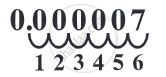# Use of Exponents to Express Small Numbers in Standard Form:

• Step 1: First of all count the number of digits from the decimal point to the last digit.
• Step 2: If there is only one digit after the zeros then simply write down that digit. Place a multiplication sign and write down the counted digits with a negative sign as the exponent to base number 10.
• Step 3: If there are two or more non-zero digits at the end of the number. Then, write down the digits followed by a decimal point after the first digit and the other non-zero digits.
• Step 4: Now calculate the number of digits in the first step and minus the number of digits appearing after the decimal point.
• Step 5: Place a multiplication sign and write down the counted digits with a negative sign as an exponent to base number 10.While converting a very very small number like 0.000007, we need to move the decimal places to the right in order to represent the number in its standard form. When being shifted to the right, the exponent will be negative.

#### Example

Express the following number in standard form.

0.000035

0.000035 = 3.5 × 10– 5

#### Example

Express the following number in standard form.

4050000

4050000 = 4.05 × 106

#### Example

Express the following number in the usual form.

3.52 × 105
3.52 × 105 = 3.52 × 100000 = 352000

#### Example

Express the following number in the usual form.

7.54 × 10– 4
7.54 × 10– 4 = 7.54/10^4 = 7.54/10000 = 0.000754.

#### Example

Express the following numbers in the usual form.

3 × 10– 5
3 × 10– 5 = 3/10^5 = 3/100000 = 0.00003.
If you would like to contribute notes or other learning material, please submit them using the button below.

### Shaalaa.com

How to Express the Numbers in the Standard form? [00:14:30]
S
0%